# SSC CGL Tier 1 Exam Question Paper For held on 10 September 2016 Reasoning

## SSC CGL Tier 1 Exam Question Paper For held on 10 September 2016 Reasoning

SSC CGL Tier 1 Exam Question Paper For held on 10 September 2016 Reasoning

EXAM DATE : 10 September 2016

EXAM NAME : SSC Examination 2016

TOTAL MARKS : 200

Question 1.Select the related word/letters/numbers from the given alternatives:

Cytology : Cells :: ? : Birds

Options:

1) Odontology
2) Mycology
3) Etymology
4) Ornithology

Question 2.Select the related word/letters/numbers from the given alternatives:

RIGT : WDBY :: FUSH : ?

Options:

1) PKJQ
2) BYWD
3) DXWB
4) QKJR

Question 3.Select the related word/letters/numbers from the given alternatives:

42 : 56 :: 110 : ?

Options:

1) 18
2) 132
3) 136
4) 140

Question 4. For the following questions

Find the odd word/letters/number from the given alternatives.

Options:

1) Rupee
2) Pound
3) Yen
4) Currency

Question 5. For the following questions

Find the odd word/letters/number from the given alternatives.

Options:

1) DAEH
2) KIMP
3) HEIL
4) FCGJ

Question 6. For the following questions

Find the odd word/letters/number from the given alternatives.

Options:

1) 436
2) 284
3) 507
4) 365

Question 7.Arrange the following words as per order in the dictionary

1. Direction 2. Directed 3. Director 4.Directing

Options:

1) 1, 4, 3, 2
2) 2, 4, 1, 3
3) 4, 2, 3, 1
4) 4, 1, 2, 3

Correct Answer: 2, 4, 1, 3

Question 8.A series is given, with one term missing. Choose the correct alternative from the given ones that will complete the series.

IGT, JHS, KIR, LJQ, MKP, ?

Options:

1) LOP
2) NOP
3) NLO
4) LNO

Question 9.A series is given, with one term missing. Choose the correct alternative from the given ones that will complete the series.

6, 10, 18, 34, ?, 130

Options:

1) 78
2) 52
3) 66
4) 94

Question 10.Introducing Reeta, Monica said, “She is the only daughter of my father’s only daughter.” How is Monica related to Reeta?

Options:

1) Aunt
2) Niece
3) Cousin
4) Mother

Question 11.In a row of students, if John , who is 16th from the left, and Johnson, who is 8 from the right, interchange their positions, John becomes 33rd from left. How many students are there in a row?

Options:

1) 38
2) 39
3) 40
4) 41

Question 12.From the given alternative words, select the word which cannot be formed using the letters of the given word:

Options:

1) MISSION
2) DISMISS
3) MASONS

Question 13.If in a certain language TEACHER is coded as QBXZEBO, then how is STUDENT coded in the same language?

Options:

1) PQRBAQK
2) PQRABKQ
3) PQRKBAQ
4) PRKQBAQ

Question 14.If ‘x’ means addition, ” means division, ‘÷’ means subtraction and ‘+’ means multiplication, then which of the equations is correct?

Options:

1) 16 x 5 ÷ 10 + 4 3= 19
2) 16 + 5 ÷ 10 x 4 3 = 9
3) 16 + 5 10 x 4 ÷ 3 = 9
4) 16 5 x 10 ÷ 4 + 3 = 12

Correct Answer: 16 + 5 10 x 4 ÷ 3 = 9

Question 15.If 4 x 5 x 2 = 524, 3 x 7 x 2 = 723 and 6 x 8 x 7 = 876 then 9 x 4 x 5 = ?

Options:

1) 495
2) 459
3) 549
4) 954

Question 16.Select the missing numbers from the given responses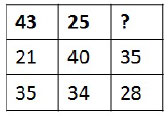Options:

1) 36
2) 46
3) 40
4) 26

Question 17.Johnson left for his office in his car. He drove 15km towards North and then 10km towards West. He then turned to the South and covered 5km. Further he turned to East & moved 8km. Finally he turned right and moved 10km. How far & in which direction is he from his starting point?

Options:

1) 2 km, West
2) 5 km, East
3) 3 km, North
4) 3 km, South

Question 18.Consider the given statement/s to be true and decide which of the given conclusions/assumptions can definitely be drawn from the given statement.

Statements:

1. All stenographers are lazy.
2. Some men are stenographers.

Conclusions:

I. All lazy people are men.
II. Some men are lazy.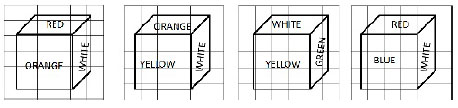Options:

1) Only conclusion I follows
2) Only conclusion II follows
3) Both conclusion I and conclusion II follow
4) Neither conclusion I nor conclusion II follows

Correct Answer: Only conclusion II follows

Question 19.Four positions of a cube are shown below. Which color is opposite to white color in the given cubes?

Options:

1) Orange
2) Blul
3) Red
4) Yellow

Question 20.Which one of the following figures best represents the relationship among Kitchen, House and Garden?

Options: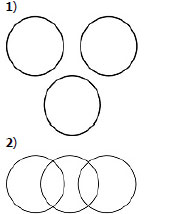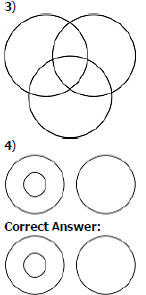Question 21.Which answer figure will complete the pattern in the question figure?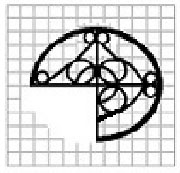Options: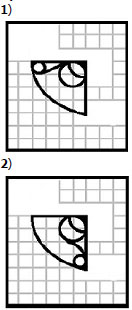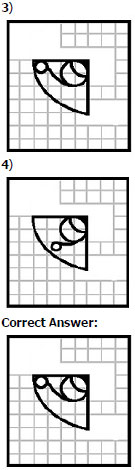Question 22.From the given answer figures, select the one in which the question figure is hidden/embedded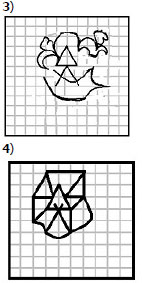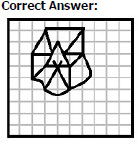Question 23.A piece of paper is folded and cut as shown below in the question figures. From the given answer figures, indicate how it will appear when opened.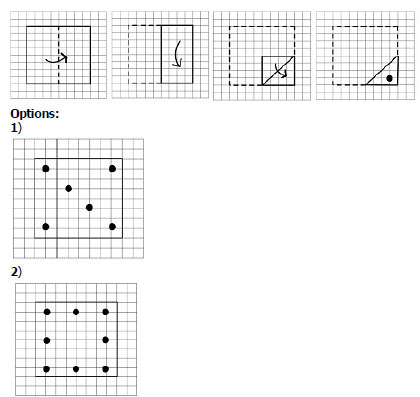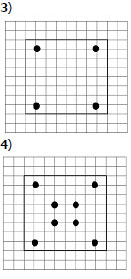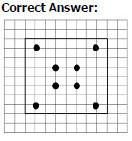Question 24.If a mirror is placed on the line MN, then which of the answer figures is the right image of the given figure?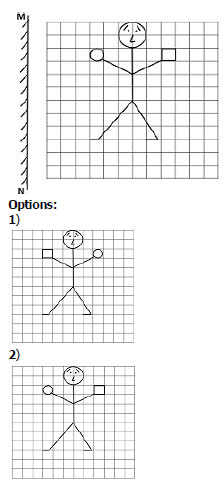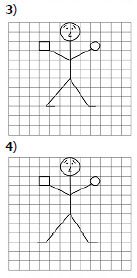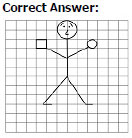Question 25.In the question, a word is represented by only one set of numbers as given in any one of the alternatives. The sets of numbers given in the alternatives are represented by two classes of alphabets as in two matrices given below. The columns and rows of Matrix I are numbered from 0 to 4 and that of Matrix II are numbered from 5 to 9. A letter from these matrices can be represented first by its row and next by its column, e.g., ‘P’ can be represented by 02, 13, etc., and ‘A’ can be represented by 57, 68, etc. Similarly you have to identify the set for the word ‘GUNS’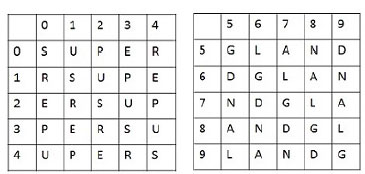Options:

1) 88, 23, 59, 33
2) 66, 40, 67, 11
3) 55, 34, 77, 44
4) 99, 12, 86, 22

Correct Answer: 99, 12, 86, 22

Scroll to Top